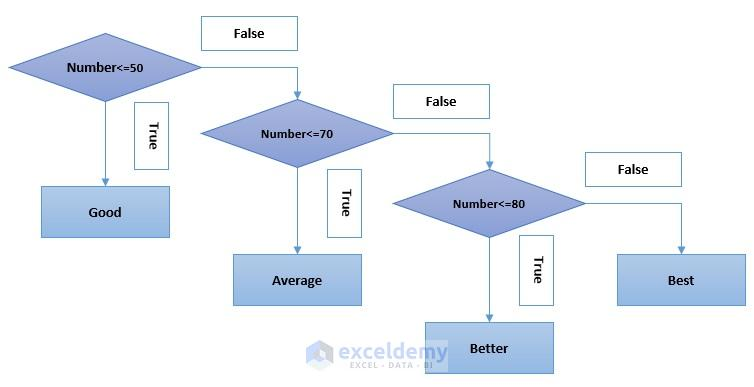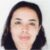Disclosure: This post may contain affiliate links, meaning when you click the links and make a purchase, we receive a commission.

# Excel If Function with Multiple Conditions (Nested IF)

We have already seen, how to use the IF function in basic Excel formulas. Using the IF with other functions together, in a complex formula, allows you to test multiple conditions and criteria. In this article, we are going to analyze Excel If function multiple conditions use.

When you will be doing some complex data analysis, you might be needed to analyze more than one conditions at a time. It means you might have to make a complex Excel formula that will test multiple conditions.

In this article, I will make several Excel formulas that will clear the whole idea.

So, stay tuned…

## Evaluation of Nested If Functions in Excel

Look at the below example where we stated some results based on some conditions. Here the formula which is written below the table is used for cell C2. This formulated cell was dragged down for getting the result for the whole column.The above example follows the nested if functions. The nested if functions in excel follow some rules. As the name If defines condition so it will look for a certain condition. If a certain condition is achieved then it will give us a value. If the condition is not achieved then it will look for another defined result. In nested if functions, we work with so many ifs. While defining results in a certain cell the defined formula for that specific cell will look for the exact condition. If the condition matches then you will get the result.

Things are more complicated while working with numbers. In nested if functions you have to be very careful while defining conditions as the same conditions can be applied to other numbers too. Like number 49 is less than 50 and 80. If you have a condition for both number 50 and number 80 then you might get an accurate result. So proper nesting is very important and it has to be maintained from either lowest number to highest number or highest number to lowest number. Observe the flowchart below for better understanding the evaluation.

Follow the following flowchart for the example stated above for getting a proper idea of how the nested if functions work.We are showing the above Excel formula in this flowchart. The formula is easy to read from this flowchart. When you will make complex formulas, you can think in this way.

## Grade Calculation in Excel with Nested if Function

Let`s say we have a number of students who obtained some specific numbers in some specific subjects. We want to convert these numbers into the equivalent grade. The grades with the number range are given. For this, we can use the nested if function. The procedure is very simple and it is given below.

• Initially, our table looks like the picture below where we want to obtain the equivalent grades.• Write this formula in cell C2 and drag this formulated cell to the rest of Column C. By doing this you will get the result for the whole column.
``````=IF(B2<50,"F",
IF(B2<60,"D",
IF(B2<65,"D+",
IF(B2<70,"C",
IF(B2<75,"C+",
IF(B2<80,"B",
IF(B2<85,"B+",
IF(B2<90,"A","A+"))))))))``````Note: Maintain proper order while defining conditions (lowest to highest/highest to lowest)

## If Function with 3 Conditions

Suppose you want to allocate some number of students in the thesis/project program. The conditions are,

Condition 1: The student has to obtain a CGPA of more than 2.50 (must be fulfilled)

And Condition 2: He has to earn a total number of credits more than or equal to 110, Or Condition 3: He has to complete at least 1 elective course

Here, A student must fulfill the 1st condition. After fulfilling the 1st condition, either the 2nd or 3rd condition needs to be fulfilled to be eligible to take the thesis/project.Now. write the below formula in Cell E2 and copy this formulated cell to the rest of column E where you want to find your result.

``=IF(AND(B2>=2.5,OR(C2>=110,D2>=1)), "Yes","No")``

By doing this you will get the desired result that you were looking for. The result is shown below.## Multiple If And Statements in Excel

Let`s work on an example where a patient with HIV/AIDS and the opportunistic disease Tuberculosis would first be sent to Ward One, to collect ARVS (Antiretrovirals) and then to the respiratory department of the hospital, to receive treatment and palliative care for Tuberculosis. A patient with HIV/AIDS and the opportunistic disease Kaposi’s sarcoma would first be sent to Ward One to collect ARVS (Antiretrovirals) and then to the oncology department of the hospital, to receive treatment for Kaposi’s sarcoma.

A patient with HIV/AIDS and cardiovascular disease would first be sent to the Ward One to collect ARVS (Antiretrovirals) and then to the cardiology department of the hospital, to receive treatment for cardiovascular disease. If the patient does not have AIDS, but one of the other opportunistic infections only or another disease, they bypass Ward One and go directly to the department they need.

The source data is shown below:1) We now are going to use the IF and AND functions in one complex formula, in order to determine the route each respective patient must take in the hospital.

2) In Cell D5 type the following formula:

``= IF(AND(B5="Yes",C5="Tuberculosis"), "Patient sent to Ward One, then respiratory department",` `IF(AND(B5="Yes",C5="Kaposi's sarcoma"),"Patient sent to Ward One, then oncology department",` `IF(AND(B5="Yes",C5="Cardiovascular disease"),"Patient sent to Ward One, then cardiology department", "Bypass Ward One and go directly to department")))``3) Press CTRL-ENTER to get the formula to deliver the route needed, given the conditions in Cell B5 and C5 that the formula is using for evaluation. We see that if the patient has HIV/AIDS and the opportunistic infection Tuberculosis, this patient must first go to Ward One and then to the respiratory department of the hospital. The formula has, therefore, delivered the correct result.4) Then double-click and send the formula down, since we used relative references, the formula will copy down correctly as shown below.5) And there you have it, the correct route for each patient registered is given by the formula.

## Things to Remember While Working With Multiple IFs

You need to be aware of the following things while working with multiple if functions.

1. In Excel 2007 – 2016, total of 64 conditions can be nested up while working with multiple ifs.
2. You have to maintain a proper order while working with multiple ifs.
3. If your formula contains too many ifs, it’s better to use OR and AND functions with that. The OR and AND functions usually reduce the formula size. You can also use the  CONCATENATE function. If necessary, VLOOKUP, LOOKUP, INDEX/MATCH these functions can also be used instead of multiple functions.

## Conclusion

If you have multiple criteria for different situations, it’s often best to combine logical functions. Combining logical functions allows one to evaluate many conditions and deliver the needed output for the said conditions. Please feel free to comment below and tell us the highest number of criteria you have ever evaluated, using logical function combinations.

Excel If Function with Multiple Conditions (Nested IF)

## Review Section: Test your Understanding

1. What is the syntax of the IF Function?

2. What is the syntax of the AND Function?

3. What does the IF Function test?

Taryn is a Microsoft Certified Professional, who has used Office Applications such as Excel and Access extensively, in her interdisciplinary academic career and work experience. She has a background in biochemistry, Geographical Information Systems (GIS) and biofuels. She enjoys showcasing the functionality of Excel in various disciplines.

1. ReplyRAHUL SINGH Jan 30, 2017 at 2:17 PM

Hi Kawser Thanks for the blog.

I would like to make an adjustment herein . When the HIV/AIDS Condition is “No” in your sheet , the patient is requested to “Bypass Ward One and go directly to department”. Here, the name of the specific department is not mentioned (respiratory, cardiology or otherwise).

However if you modify the formula as follows, the patient is requested to bypass ward one and go to the specific department (respiratory, cardiology , Emphysema treatment):

=IF(AND(B5=”Yes”,C5=”Tuberculosis”),”Patient sent to Ward One, then respiratory department”,IF(AND(B5=”Yes”,C5=”Kaposi’s sarcoma”),”Patient sent to Ward One, then oncology department”,IF(AND(B5=”Yes”,C5=”Cardiovascular disease”),”Patient sent to Ward One, then cardiology department”,IF(AND(B5=”No”,C5=”Tuberculosis”),”By Pass ward one and go directly to respiratory department”,IF(AND(B5=”No”,C5=”Emphysema”),”Bypass Ward One and go directly to Emphysema treatment department”,IF(AND(B5=”No”,C5=”Kaposi’s sarcoma”),”Bypass ward one and go directly to oncology department”,”Bypass Ward one and directly go to cardiology department”))))))

Hope you got it :):):):)

• ReplyJan 30, 2017 at 4:30 PM

Thank you for your contribution. The modification of the formula, you suggested, would work for additional detailed routes that the hospital in question, may feel they need to add.

We can lead on from this and design an Excel Sheet with VBA using Nested IFS and a small picture of the routes, for the ten most common routes patients at a certain hospital need to take based on the most often noted diseases and disease combinations. So I will include the routes you specified in the Visual Basic Application.

So look out for that post.

2. ReplySyed Mohd Jahangir Jan 30, 2017 at 6:31 PM

I am putting the same formula , so the place on No HIV/AIDS, its showing FALSE.
Why???

• ReplyKawser Jan 30, 2017 at 7:36 PM

Syed,
Sometimes when the formula is posted in WordPress platform, it might be changed. Please, download the working file and check the formula there.

3. ReplyAhmad Akar Apr 24, 2018 at 1:49 PM

Dear Kawser,

Thanks for the blog.very nice and helpful.
regarding (If Function with 3 Conditions) I think the conditions should be like this:

The student has to obtain a CGPA more than 2.50.
He has to earn a total number of credits more than or equal to 110
He has to complete at least 1 elective courses

The student must fulfill the three conditions, not just the 1st and 2nd, or 1st and 3rd

Am I right?

regards

• ReplyApr 24, 2018 at 2:14 PM

I have updated this part. For your clarification: the condition 1 must be fulfilled to be eligible to take the thesis work/project. Of the last conditions, students must fulfill one condition. I hope this makes things clear to you.
Best regards
Kawser

4. ReplyAhmad Akar Apr 25, 2018 at 10:33 PM

Thanks a lot Mr kawser, now it is very clear, and thanks for the update.

5. ReplyAhmad Akar Apr 26, 2018 at 12:10 PM

Thanks a lot Mr. Kawer, now it is very clear.
also, thanks for the update.

regards